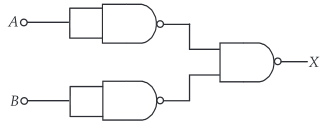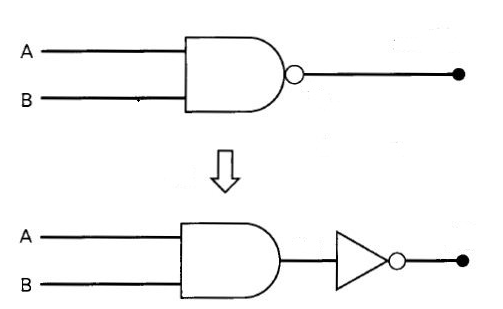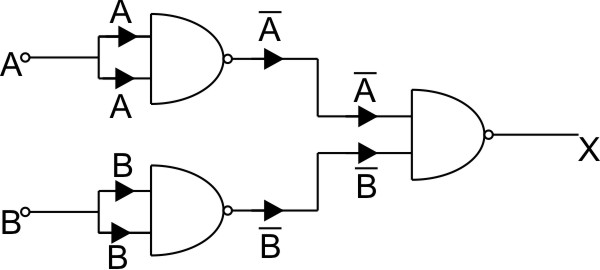#### The combination of gates shown below yieldsOption 1) NAND gate Option 2) OR gate Option 3) NOT gate Option 4) XOR gateAs we learnt in

NAND gate -

NOT + AND gate- whereinA and B are input

Y is out put(From D'morgan law)

Hence, This circuit represent OR gate.

Correct optio is 2.

Option 1)

NAND gate

This is an incorrect option.

Option 2)

OR gate

This is the correct option.

Option 3)

NOT gate

This is an incorrect option.

Option 4)

XOR gate

This is an incorrect option.

##### Posted by